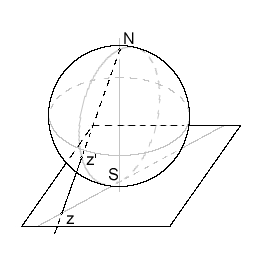# More on the Riemann sphere

In my previous discussion on the Riemann Sphere I talked of it as a closed sphere of infinite extent, but it can also be represented as a sphere of finite extent.Display of complex number on the Riemann sphere. (Photo credit: Wikipedia)

In this case imagine a sphere centred on the origin and of unit radius. Then any complex number can be represented as a point on the sphere’s surface by simply drawing a line from the pole to that point on the (complex) plane – where the line intersects the sphere is the point that represents the number (numbers of magnitude of less than one are represented by the underside of the sphere).

This is, in one sense, a consequence of the infinity of the continuum – we are projecting an infinite line on to a finite line, but as the points on both lines have the same cardinality there can be a one to one correspondence – it’s another thing of beauty, I think.

Advertisements